Question

# Modulus of Elasticity, Stress and Strain

Refer to the diagram below. Determine the total elongation of the strut AB due to the weight of W = 14 kN. Assume the strut has a diameter of 10 mm and is made of steel with an E = 200,000 MPa.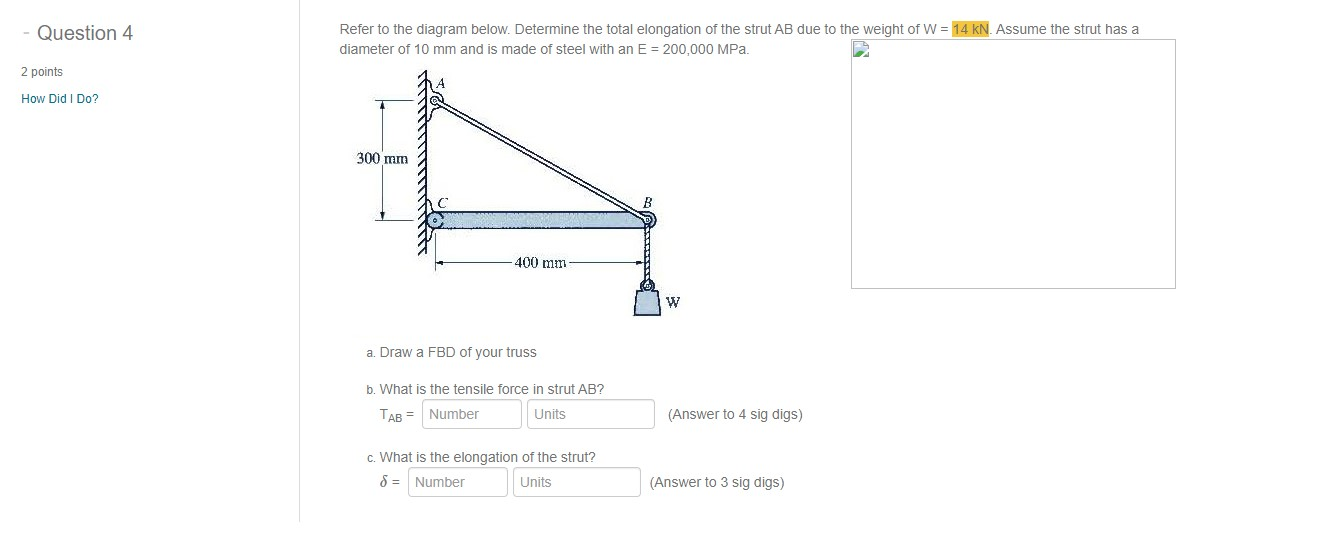Dear student, you can refer to the question https://www.homeworklib.com/answers/1935541/refer-to-fig-p10-6-determine-the-total-elongation

#### Earn Coins

Coins can be redeemed for fabulous gifts.

Similar Homework Help Questions
• ### Refer to Fig. P10-6. Determine the total elongation of strut AB due to a weight W=...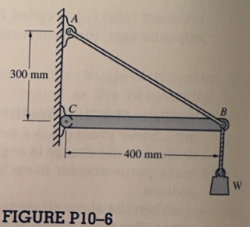Refer to Fig. P10-6. Determine the total elongation of strut AB due to a weight W= 15 kN if the strut has a 10-mm diameter and is made of 300 mm steel with E 210 GPa.

• ### Modulus of Elasticity, Stress and Strain Elongation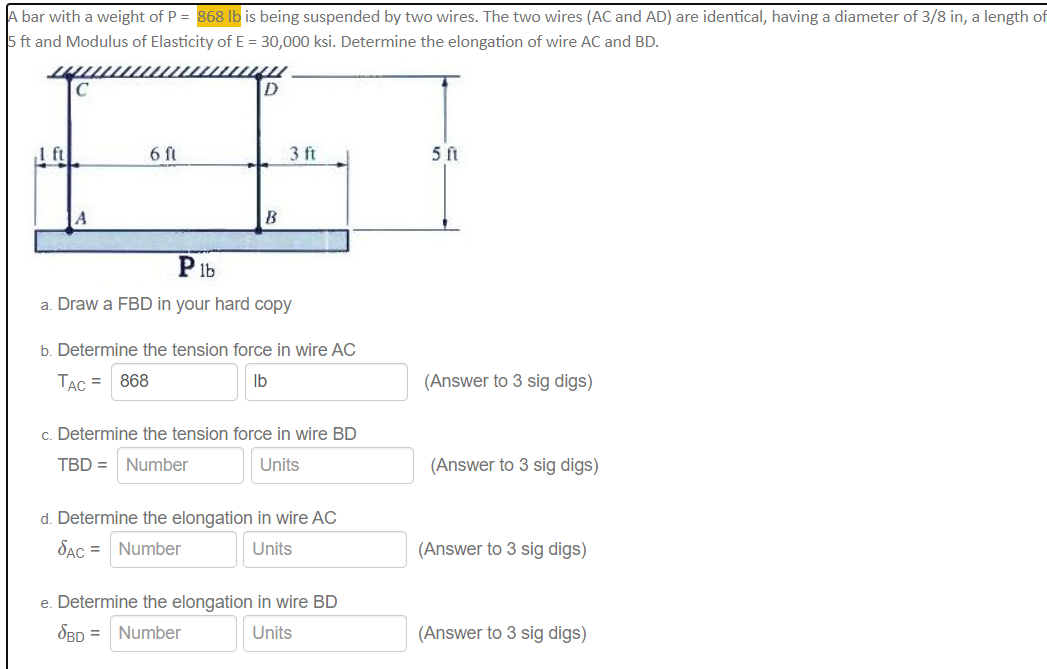A bar with a weight of P =  868 lb is being suspended by two wires. The two wires (AC and AD) are identical, having a diameter of 3/8 in, a length of 5 ft and Modulus of Elasticity of E = 30,000 ksi. Determine the elongation of wire AC and BD.

• ### Plz show in graph paper if possible. Thanks :) find stress, strain and draw the graph....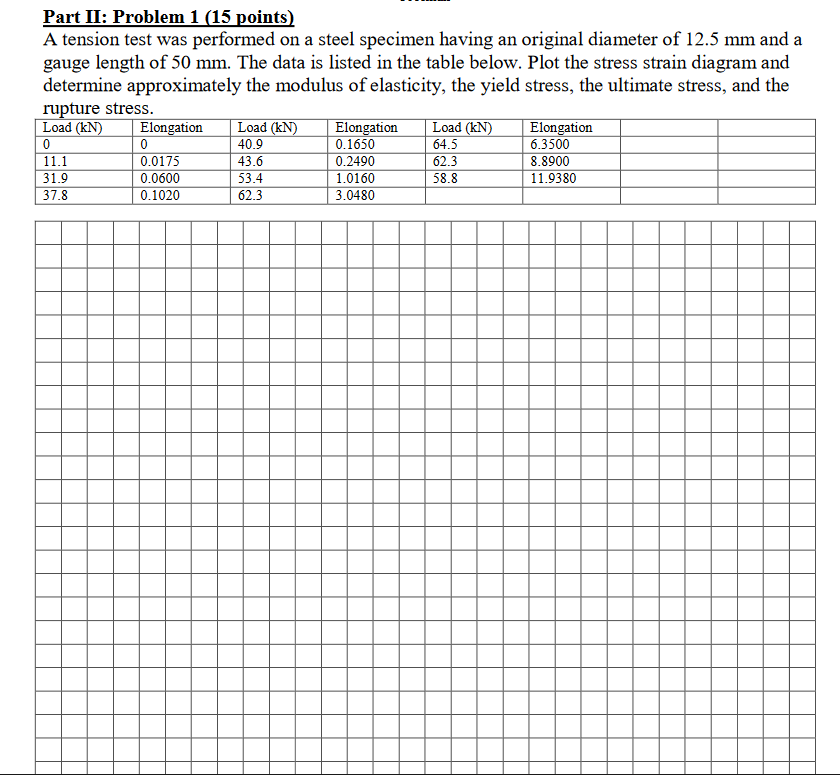Plz show in graph paper if possible. Thanks :) find stress, strain and draw the graph. if it is blurry. plz zoom Part II: Problem 1 (15 points) A tension test was performed on a steel specimen having an original diameter of 12.5 mm and a gauge length of 50 mm. The data is listed in the table below. Plot the stress strain diagram and determine approximately the modulus of elasticity, the yield stress, the ultimate stress, and the rupture...

• ### Can anyone please solve step by step Thanks Please solve this Question don’t solve other Th...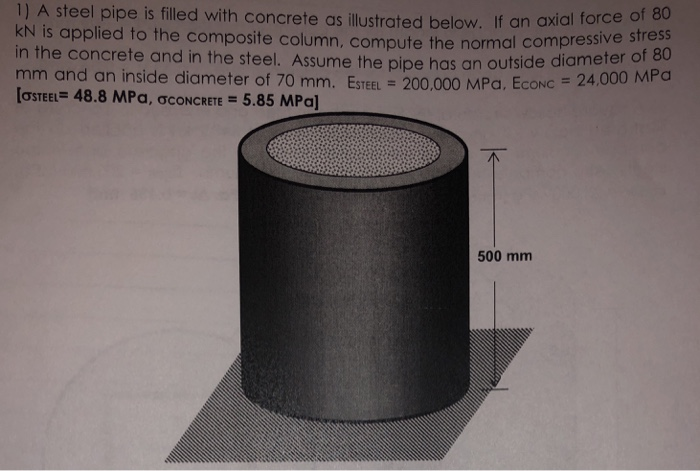can anyone please solve step by step Thanks Please solve this Question don’t solve other Thanks 1) A steel pipe is filled with concrete as ilustrated below. If an axial force of kN is applied to the composite column, compute t in the concrete and in the steel. Assume the pipe has an outsid mm and an inside diameter of 70 mm. ESTEEL 200,000 MPa, EcoNC [oSTEEL 48.8 MPa, ơCONCRETE 5.85 MPa] he normal compressive stress e diameter of 80...

• ### Axial (Normal) Stress, Strain and Deformation The system is loaded and supported as shown. The system...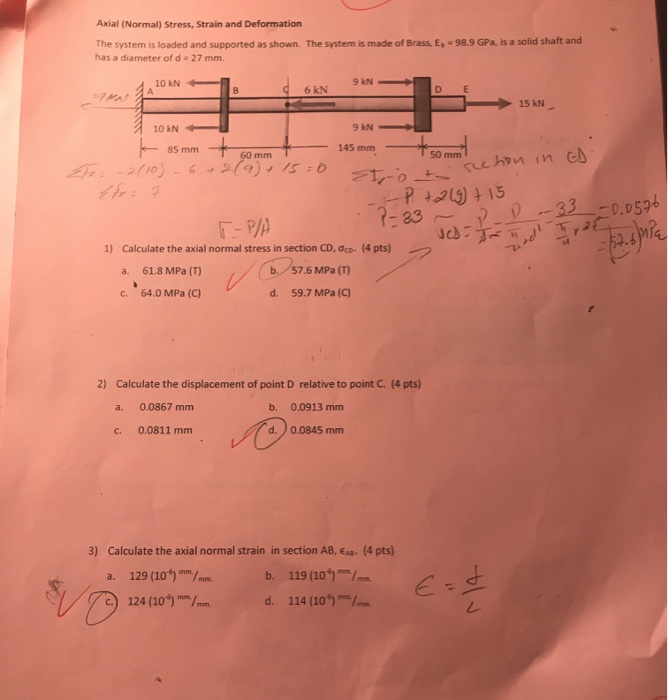Axial (Normal) Stress, Strain and Deformation The system is loaded and supported as shown. The system is made of Brass, E, 98.9 GPa, is a solid shaft and has a diameter of d 27 mm. 9 kN 10 kN A D B 6 kN E 15 kN 9 kN 10 kN + En: 2010) 629) 145 mm 85 mm 50 mm 60 mm See hon in cd P tol15 P-a3 -33-0.056 T-P/A Calculate the axial normal stress in section CD,...

• ### the modulus of elasticity is not given Problem 4.2: The mechanism below is in equilibrium for...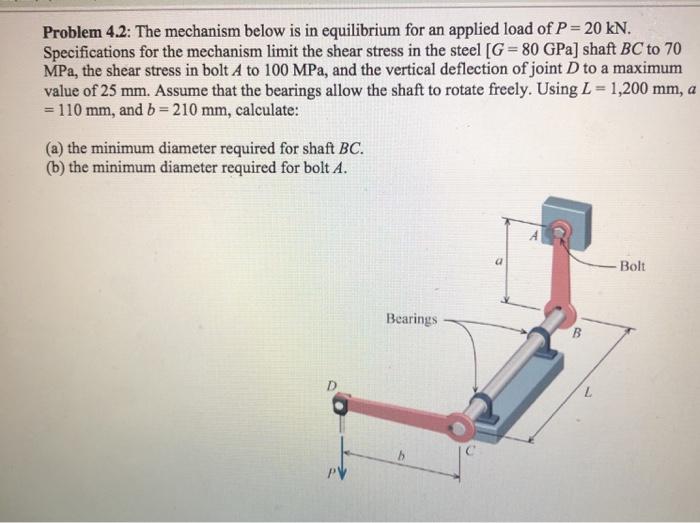the modulus of elasticity is not given Problem 4.2: The mechanism below is in equilibrium for an applied load of P = 20 kN. Specifications for the mechanism limit the shear stress in the steel [G = 80 GPa) shaft BC to 70 MPa, the shear stress in bolt A to 100 MPa, and the vertical deflection of joint D to a maximum value of 25 mm. Assume that the bearings allow the shaft to rotate freely. Using L =...

• ### A tie rod (1) and a pipe strut (2) are used to support a 50-kN load,...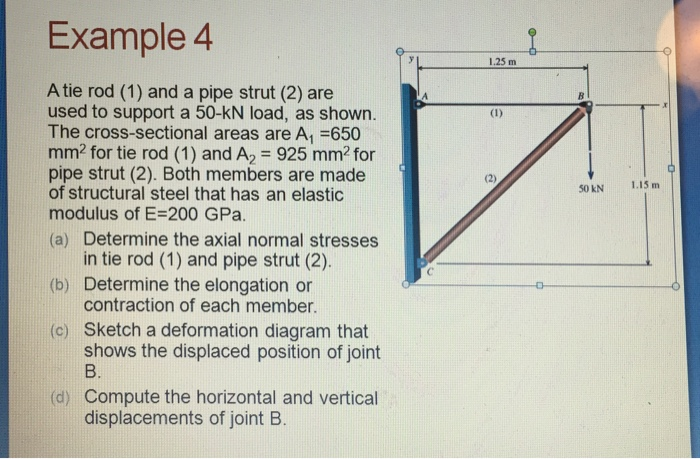A tie rod (1) and a pipe strut (2) are used to support a 50-kN load, as shown. The cross-sectional areas are A, =650 mm? for tie rod (1) and A_2 = 925 mm? for pipe strut (2). Both members are made of structural steel that has an elastic modulus of E = 200 GPa. (a) Determine the axial normal stresses in tie rod (1) and pipe strut (2). (b) Determine the elongation or contraction of each member. (c) Sketch...

• ### The assembly shown in the figure below consists of solid AB, BC and CD rods connected...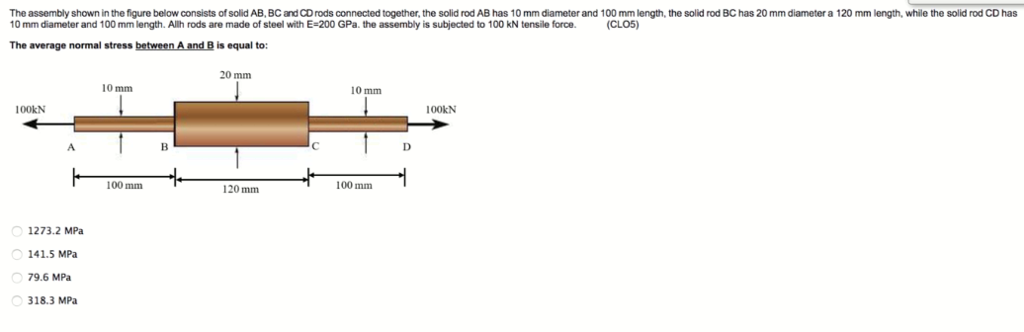The assembly shown in the figure below consists of solid AB, BC and CD rods connected together, the solid rod AB has 10 mm diameter and 100 mm length, the solid rod BC has 20 mm diameter a 120 mm length, while the solid rod CD has 10 mm diameter and 100 mm length. Allh rods are made of steel with E-200 GPa. the assembly is subjected to 100 kN tensile force (CLO5) The average normal stress between A and...

• ### The rod ABCD is made of an aluminum alloy with a modulus of elasticity E = 70 GPa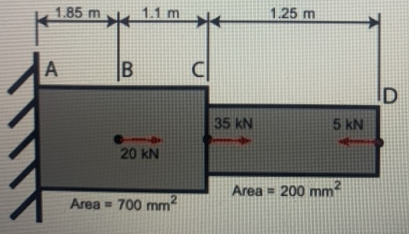The rod ABCD is made of an aluminum alloy with a modulus of elasticity E = 70 GPa. For the loading shown, and neglecting the weight of the rod, determine the stress in sections AB, BC, and CD and the deflection of point D. For stress, answer to nearest whole number. Use 3 decimal places for displacement. Drawing is not to scale. Assume compressive stresses are positive, and that displacement to the right is positive. Stress in AB = _______ MPa Stress...

• ### A tie rod (1) and a pipe strut (2) are used to support a 50-kN load,...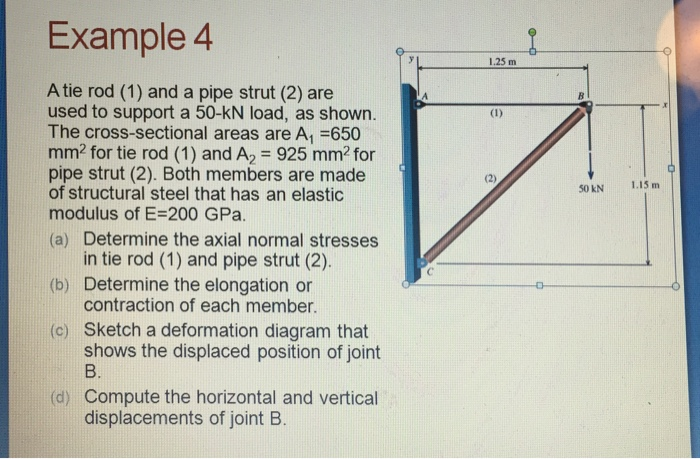A tie rod (1) and a pipe strut (2) are used to support a 50-kN load, as shown. The cross-sectional areas are A1 =650 mm2 for tie rod (1) and A2 = 925 mm2 for pipe strut (2). Both members are made of structural steel that has an elastic modulus of E=200 GPa. a) Determine the axial normal stresses in tie rod (1) and pipe strut (2). b) Determine the elongation or contraction of each member. c) Sketch a deformation...